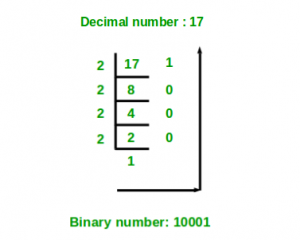# Python program to convert decimal to binary number

Given a decimal number as input, the task is to write a Python program to convert the given decimal number into equivalent binary number.

Examples :

```Input : 7
Output :111

Input :10
Output :1010```

Method #1: Recursive solution

```DecimalToBinary(num):
if num > 1:
DecimalToBinary(num // 2)
print num % 2```Below is the implementation of above recursive solution:

 `# Function to convert decimal number ` `# to binary using recursion ` `def` `DecimalToBinary(num): ` `     `  `    ``if` `num > ``1``: ` `        ``DecimalToBinary(num ``/``/` `2``) ` `    ``print``(num ``%` `2``, end ``=` `'') ` ` `  `# Driver Code ` `if` `__name__ ``=``=` `'__main__'``: ` `     `  `    ``# decimal value ` `    ``dec_val ``=` `24` `     `  `    ``# Calling function ` `    ``DecimalToBinary(dec_val) `

Output:

```11000
```

Method #2: Decimal to binary using in-built function

 `# Python program to convert decimal to binary ` `   `  `# Function to convert Decimal number  ` `# to Binary number  ` `def` `decimalToBinary(n):  ` `    ``return` `bin``(n).replace(``"0b"``, "")  ` `   `  `# Driver code  ` `if` `__name__ ``=``=` `'__main__'``:  ` `    ``print``(decimalToBinary(``8``))  ` `    ``print``(decimalToBinary(``18``))  ` `    ``print``(decimalToBinary(``7``))  `

Output:

```1000
10010
111
```

My Personal Notes arrow_drop_upCheck out this Author's contributed articles.

If you like GeeksforGeeks and would like to contribute, you can also write an article using contribute.geeksforgeeks.org or mail your article to contribute@geeksforgeeks.org. See your article appearing on the GeeksforGeeks main page and help other Geeks.

Please Improve this article if you find anything incorrect by clicking on the "Improve Article" button below.

Improved By : nidhi_biet

Article Tags :

1

Please write to us at contribute@geeksforgeeks.org to report any issue with the above content.